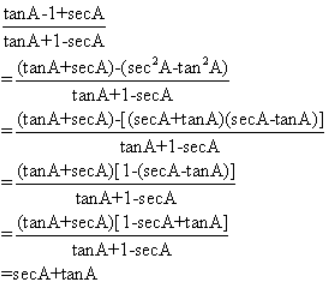×#### Thank you for registering.

One of our academic counsellors will contact you within 1 working day.

Click to Chat

1800-1023-196

+91-120-4616500

CART 0

• 0

MY CART (5)

Use Coupon: CART20 and get 20% off on all online Study Material

ITEM
DETAILS
MRP
DISCOUNT
FINAL PRICE
Total Price: Rs.

There are no items in this cart.
Continue Shopping
```
Prove that secA+ tanA-1÷ tanA- secA+1= cosA÷1- sinA
Prove that  secA+ tanA-1÷ tanA- secA+1= cosA÷1- sinA

```
3 years ago

```= 1+sinA    cosA= 1+sinAx(1-sinA)    cosAx(1-sinA)=      1-sin2A          cosA(1-sinA)=       cos2A              cosA(1-sinA)=     cosA          1-sinAHence Proved
```
3 years ago
```							Multiply ((tanA-1)+(secA))/ ((tanA+1)-secA)) by ((tanA-1)+(secA)) on numerator and denominator. Then use the identitype a2-b2 to proceed further. Thanks.
```
2 years ago
```							Dear Student,Please find below the solution to your problem.secA+tanA-1)/tanA-secA+1)We know that sec^2-tan^2=1=(secA+tanA-(sec^2 A - tan ^2A)) /tanA-secA+1)We also know that a^2-b^2=(a+b)(a-b)= (sec A + tan A - (sec A + tan A) ( sec A - tan A)) / ( tanA-secA+1)= ( sec A + tan A ) ( 1- (sec A - tan A)/ ( tanA-secA+1)= (sec A + tan A)/(+ tan A - sec A)/(tan A- sec A+ 1)= (sec A + tan A)= 1/cos A + sin A/ cos A= (1+ sin A)/ cos A= (1 + sin A )(1- sin A)/(cos A (1- sin A))= (1- sin ^2 A/(cos A (1- sin A))= cos ^2 A / (cos A (1- sin A))= cos A /(1- sin A)Thanks and Regards
```
6 months ago
Think You Can Provide A Better Answer ?

## Other Related Questions on Trigonometry

View all Questions »### Course Features

• 731 Video Lectures
• Revision Notes
• Previous Year Papers
• Mind Map
• Study Planner
• NCERT Solutions
• Discussion Forum
• Test paper with Video Solution### Course Features

• 31 Video Lectures
• Revision Notes
• Test paper with Video Solution
• Mind Map
• Study Planner
• NCERT Solutions
• Discussion Forum
• Previous Year Exam Questions